# Buckling Analysis Control

Enter the load cases and related data for the buckling analysis of a structure.

midas Civil follows the procedure noted below for buckling analysis.

1. A buckling analysis process requires static analysis results to formulate the initial geometric stiffness matrix. Accordingly, enter the load cases to be applicable for the buckling analysis following the procedure for static analysis.

2. Invoke the dialog box of the Analysis > Analysis Control > Buckling Analysis Control menu and enter the required number of modes and data necessary for convergence and assign the load combination to be applied to the buckling analysis.

3. Select the Analysis > Perform Analysis menu or click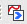Perform Analysis to carry out the analysis.

4. Once the analysis is successfully completed, use either the Results > Buckling Mode Shapes function or the Results > Result Tables > Bucking Mode Shape function to review the buckling mode shapes and critical load factors for each mode shape.

5. To calculate buckling loads, enter the self-weight (Constant) and additional loads (Variable) and then perform the analysis. The Eigenvalue resulting from the analysis is the critical load factor. Accordingly, the buckling load = critical load factor× additional load (Variable) + self-weight (Constant).

From the Main Menu select Analysis >  Analysis Control > Buckling.

####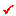Buckling Modes

Number of Modes: Enter the number of buckling modes.

Positive Value Only: Produce the eigenvalue results only for the loading direction.

Note
Once buckling analysis is performed, buckling modes are computed for both the loading direction and the opposite direction. However, for static loads whose loading direction is fixed, it is not necessary to compute the buckling mode shape and factor for the opposite direction.

####Buckling Modes

Number of Modes: Enter the number of buckling modes.

Positive Value Only: Produce the eigenvalue results only for the loading direction.

Note
Once buckling analysis is performed, buckling modes are computed for both the loading direction and the opposite direction. However, for static loads whose loading direction is fixed, it is not necessary to compute the buckling mode shape and factor for the opposite direction.

Search: Calculate the buckling load factor within the defined range.

From: Minimum value of the buckling load factor

To: Maximum value of the buckling load factor

Note

If the same values are entered in the 'From' and 'To' field, the buckling load factors closest to the entered value are calculated.

Sturm Sequence Check: Check to detect any missed buckling load factors. If there are any missed buckling load factors, an error message is displayed in the message window.Frame Geometric Stiffness Option

Option for formulating the geometric stiffness matrix in a frame member

Consider Axial Force Only : Consider axial force only when formulating the geometric stiffness matrix.

####Buckling Combination

Enter the load cases for the buckling analysis. Different Load Cases with the corresponding Scale Factors may be entered.

Variable: Consider increase or decrease in loads (live loads, etc.)

Constant: Do not consider increase or decrease in loads (self-weight, dead loads)

Note
Strictly speaking, Constant should contain only the self-weight of the structure. However, it is common to apply dead load to slab, along with the self-weight. Accordingly, dead load can be entered as Constant Type.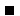To enter a new or additional load case

Enter the Load Case and Scale Factor; then click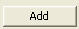.To modify the scale factor for a previously entered load case

Select the load case, modify the Scale Factor and click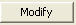.To delete a previously entered load case

Select the load case to be deleted from the load case list at the bottom of the dialog box and click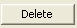.

Buckling Analysis may not be carried out in conjunction with P-Delta Analysis or Eigenvalue Analysis. Buckling Analysis is limited to truss element, beam element (including tapered beam element) and plate element.

To delete all the entered data, click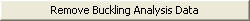.Revision of Civil 2015 (v1.1)